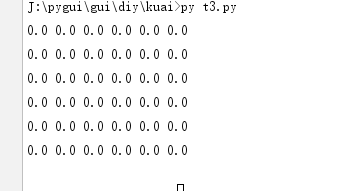# python numpy.array 转txt

2019-09-25 python 1233
```import numpy as np

a = np.zeros((6, 6))
txt = []
for y in a:
txt.append(" ".join([str(x) for x in y]))

content = "\n".join(txt)
with open("a.txt", "w+", encoding="utf-8") as f:
f.write(content)

print(content)```0条评论